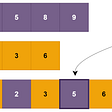# Leetcode

21. Merge Two Sorted Lists

Merge two sorted linked lists and return it as a sorted list. The list should be made by splicing together the nodes of the first two lists.

Example 1:

`Input: l1 = [1,2,4], l2 = [1,3,4]Output: [1,1,2,3,4,4]`

Example 2:

`Input: l1 = [], l2 = []Output: []`

Example 3:

`Input: l1 = [], l2 = Output: `

Constraints:

• The number of nodes in both lists is in the range `[0, 50]`.
• `-100 <= Node.val <= 100`
• Both `l1` and `l2` are sorted in non-decreasing order.

Solution1: Iteratively

`class Solution:    def mergeTwoLists1(self, l1, l2):        dummy = cur = ListNode(0)        while l1 and l2:            if l1.val < l2.val:                cur.next = l1                l1 = l1.next            else:                cur.next = l2                l2 = l2.next            cur = cur.next        cur.next = l1 or l2        return dummy.next# TC: O(m+n), where m, n are the lengths of the two linked lists# SC: O(1)            `

Solution2: Recursively

`class Solution:    def mergeTwoLists2(self, l1, l2):        if not l1 or not l2:            return l1 or l2        if l1.val < l2.val:            l1.next = self.mergeTwoLists2(l1.next, l2)            return l1        else:             l2.next = self.mergeTwoLists2(l1, l2.next)             return l2# TC: O(m+n)# SC: O(m+n)`

Solution3:in-place Iteratively

`class Solution:    def mergeTwoLists(self, l1, l2):        if None in (l1, l2):            return l1 or l2        dummy = cur = ListNode(0)        dummy.next = l1        while l1 and l2:           if l1.val < l2.val:               l1 = l1.next           else:                nxt = cur.next                cur.next = l2                tmp = l2.next                l2.next = nxt                l2 = tmp            cur = cur.next        cur.next = l1 or l2        return dummy.next# TC: O(m+n)# SC: O(1)`

--

--

--

## More from JEN-LI CHEN IN DATA SCIENCE

My homepage to record my thought processes for solving SQL and Algorithm questions

## How to configure express with Github Auth0## @Novax_official## JavaScript Promises 101## Decouple Your Logic From UI By Creating Your Own React Hooks## How Much Does it Cost to Build a Language Support App Like a Voice Translator?## How to increase cohesion in your Angular Application## Jen-Li Chen

In love with telling stories with data

## Knight Probability in Chessboard Solution | LeetCode-688: Medium | JavaScript Implementation## Logarithmic Algorithm for Finding Median of Two Sorted Arrays | Coding Interview